Courses

# Irodov Solutions: Universal Gravitation- 2 Notes | EduRev

## JEE : Irodov Solutions: Universal Gravitation- 2 Notes | EduRev

The document Irodov Solutions: Universal Gravitation- 2 Notes | EduRev is a part of the JEE Course Physics For JEE.
All you need of JEE at this link: JEE

Q. 218. Two Earth's satellites move in a common plane along circular orbits. The orbital radius of one satellite r = 7000 km while that of the other satellite is Δr = 70 km less. What time interval separates the periodic approaches of the satellites to each other over the minimum distance?

Ans.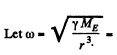circular frequency o f the satellite in the outer orbit,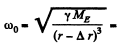ircular frequency of the satellite in the inner orbit

So, relative angular velocity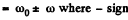is to be taken when the satellites are moving in the same sense and + sign if they are moving in opposite sense.
Hence, time between closest approaches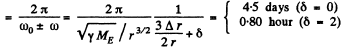where δ is 0 in the first case and 2 in the second case.

Q. 219. Calculate the ratios of the following accelerations: the acceleration w1 due to the gravitational force on the Earth's surface, the acceleration wdue to the centrifugal force of inertia on the Earth's equator, and the acceleration w3 caused by the Sun to the bodies on the Earth.

Ans.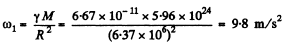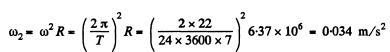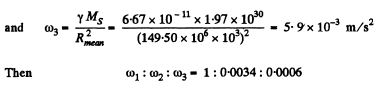Q. 220. At what height over the Earth's pole the free-fall acceleration decreases by one per cent; by half?

Ans. Let h be the sought height in the first case, so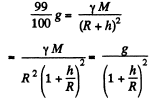or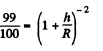From the statement of the problem, it is obvious that in this case h<<R

Thus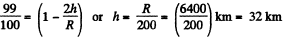In the other case if h! be the sought height, than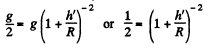From the language o f the problem, in this case h' is not very small in comparison with R. Therefore in this case we cannot use the approximation adopted in the previous case.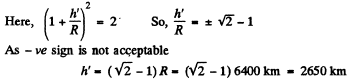Q. 221.  On the pole of the Earth a body is imparted velocity v0 directed vertically up. Knowing the radius of the Earth and the freefall acceleration on its surface, find the height to which the body will ascend. The air drag is to be neglected.

Ans. Let the mass of the body be m and let it go upto a height h.

From conservation of mechanical energy of the system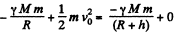Using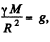in above equation and on solving we get,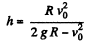Q. 222. An artificial satellite is launched into a circular orbit around the Earth with velocity v relative to the reference frame moving translationally and fixed to the Earth's rotation axis. Find the distance from the satellite to the Earth's surface. The radius of the Earth and the free-fall acceleration on its surface are supposed to be known.

Ans. Gravitational pull provides the required centripetal acceleration to the satelite. Thus if h be the sought distance, we have

so,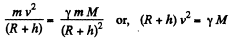or,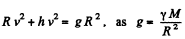Hence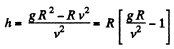Q. 223. Calculate the radius of the circular orbit of a stationary Earth's satellite, which remains motionless with respect to its surface. What are its velocity and acceleration in the inertial reference frame fixed at a given moment to the centre of the Earth?

Ans. A satellite that hovers above the earth’s equator and corotates with it moving from the west to east with the diurnal angular velocity of the earth appears stationary to an observer on the earth. It is called geostationary. For this calculation we may neglect the annual motion of the earth as well as all other influences. Then, by Newton’s law,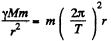where M = mass of the earth, T = 86400 seconds = period of daily rotation of the earth and r = distance of the satellite from the centre of the earth. Then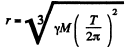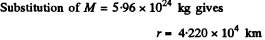The instantaneous velocity with respect to an inertial frame fixed to the centre of the earth at that moment will be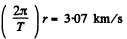and the acceleration will be the centripetal acceleration.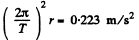Q. 224. A satellite revolving in a circular equatorial orbit of radius R = 2.00-10 km from west to east appears over a certain point at the equator every τ = 11.6 hours. Using these data, calculate the mass of the Earth. The gravitational constant is supposed to be known.

Ans. We know from the previous problem that a satellite moving west to east at a distance R = 2-00 x 104 km from the centre of the earth will be revolving round the earth with an angular velocity faster than the earth’s diurnal angualr velocity. Let

ω = angular velocity of the satellite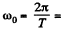anuglar velocity of the earth. Then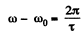as the relative angular velocity with respect to earth. Now by Newton’s law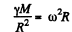So,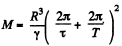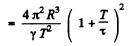Substitution gives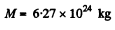Q. 225. A satellite revolves from east to west in a circular equatorial orbit of radius R = 1.00.104  km around the Earth. Find the velocity and the acceleration of the satellite in the reference frame fixed to the Earth.

Ans. The velocity of the satellite in the inertial space fixed frame is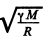east to west . With respect to the Earth fixed frame, from the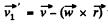the velocity is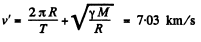Here M is the mass of the earth and T is its period of rotation about its own axis.

It would be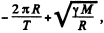if the satellite were moving from west to east

To find the acceleration we note the formula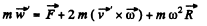Here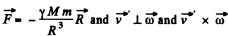is directed towards the centre of the Earth.

Thus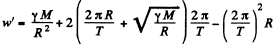toward the earth’s rotation axis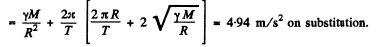Q. 226. A satellite must move in the equatorial plane of the Earth close to its surface either in the Earth's rotation direction or against it. Find how many times the kinetic energy of the satellite in the latter case exceeds that in the former case (in the reference frame fixed to the Earth).

Ans. From the well known relationship between the velocities of a particle w.r.t a space fixed fram e (K) rotating frame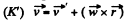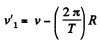Thus kinetic energy of the satellite in the earth’s frame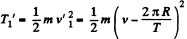Obviously when the satellite moves in opposite sense comared to the rotation of the Earth its velocity relative to the same frame would be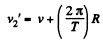And kinetic energy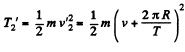(2)

From (1) and (2)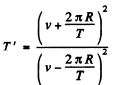(3)

Now from Newton’s second law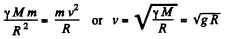(4)

Using (4) and (3).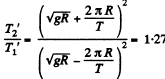nearly (Using Appendices)

Q. 227. An artificial satellite of the Moon revolves in a circular orbit whose radius exceeds the radius of the Moon η times. In the process of motion the satellite experiences a slight resistance due to cosmic dust. Assuming the resistance force to depend on the velocity of the satellite as F = αv2, where a is α constant, find how long the satellite will stay in orbit until it falls onto the Moon's surface.

Ans. For a satellite in a circular orbit about any massive body, the following relation holds between kinetic, potential & total eneigy :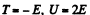(1)

Thus since total mechanical energy must decrease due to resistance of the cosmic dust, the kintetic energy will increase and the satellite will 'fair, We see then, by work eneigy theorm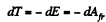So,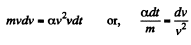Now from Netow’s law at an arbitray radius r from the moon’s centre.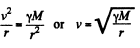(M is the mass of the moon.) Then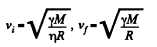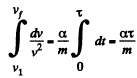or,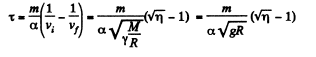where g is moon’s gravity. The averaging implied by Eq. (1) (for noncircular orbits) makes the result approximate.

Q. 228. Calculate the orbital and escape velocities for the Moon. Compare the results obtained with the corresponding velocities for the Earth.

Ans. From Newton’s second law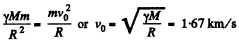(1)

From conservation of mechanical energy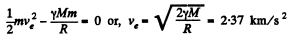(2)

In Eq. (1) and (2), M and R are the mass of the moon and its radius. In Eq. (1) if M and R represent the mass of the earth and its radius, then, using appendices, we can easily get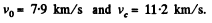Q. 229. A spaceship approaches the Moon along a parabolic trajectory which is almost tangent to the Moon's surface. At the moment of the maximum approach the brake rocket was fired for a short time interval, and the spaceship was transferred into a circular orbit of a Moon satellite. Find how the spaceship velocity modulus increased in the process of braking.

Ans. In a parabolic orbit, E = 0

So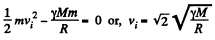where M = mass of the Moon, R = its radius. (This is just the escape velocity.) On the other hand in orbit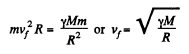Thus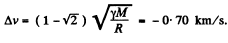Q. 230. A spaceship is launched into a circular orbit close to the Earth's surface. What additional velocity has to be imparted to the spaceship to overcome the gravitational pull?

Ans. From 1.228 for the Earth surface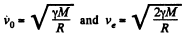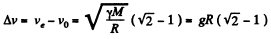This ‘kick’ in velocity must be given along the direction of motion of the satellite in its orbit

Q. 231. At what distance from the centre of the Moon is the point at which the strength of the resultant of the Earth's and Moon's gravitational fields is equal to zero? The Earth's mass is assumed to be η = 81 times that of the Moon, and the distance between the centres of these planets n = 60 times greater than the radius of the Earth R.

Ans. Let r be the sought distance, then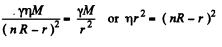or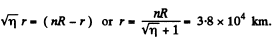Q. 232. What is the minimum work that has to be performed to bring a spaceship of mass m = 2.0.103 k g from the surface of the Earth to the Moon?

Ans. Between the earth and the moon, the potential energy of the spaceship will have a maximum at the point where the attractions of the earth and the moon balance each other. This maximum RE. is approximately zero. We can also neglect the contribution of either body to the p.E. of the spaceship sufficiently near the other body. Then the minimum energy that must be imparted to the spaceship to cross the maximum of the P.E. is clearly (using E to denote the earth)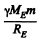With this energy the spaceship will cross over the hump in the P.E. and coast down the hill of p.E. towards the moon and crashland on it. What the problem seeks is the minimum energy reguired for softlanding. That reguies the use of rockets to loving about the braking of the spaceship and since the kinetic energy of the gases ejected from the rocket will always be positive, the total energy required for softlanding is greater than that required for crashlanding. To calculate this energy we assume that the rockets are used fairly close to the moon when the spaceship has nealy attained its terminal velocity on the moon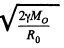where M0 is the mass of the moon and R0 is its radius. In general  dE = vdp and since the speed of the ejected gases is not less than the speed of the rocket, and momentum transfered to the ejected gases must equal the momentum of the spaceship the energy E of the gass ejected is not less than the kinetic energy of spaceship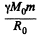Addding the two we get the minimum work done on the ejected gases to bring about the softlanding.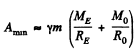On substitution we get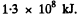Q. 233. Find approximately the third cosmic velocity v3, i.e. the minimum velocity that has to be imparted to a body relative to the Earth's surface to drive it out of the Solar system. The rotation of the Earth about its own axis is to be neglected.

Ans. Assume first that the attraction of the earth can be neglected. Then the minimum velocity, that must be imparted to the body to escape from the Sun’s pull, is, as in 1*230, equal to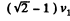where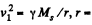radius of the earth’s orbit, Ms - mass of the Sun.

In the actual case near the earth, the pull of the Sun is small and does not change much over distances, which are several times the radius of the Earth. The velocity v3 in question is that which overcomes the earth’s pull with sufficient velocity to escape the Sun’s pull.

Thus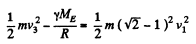where R = radius of the earth, ME = mass of the earth.

Writing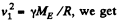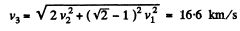Offer running on EduRev: Apply code STAYHOME200 to get INR 200 off on our premium plan EduRev Infinity!

## Physics For JEE

187 videos|516 docs|263 tests

,

,

,

,

,

,

,

,

,

,

,

,

,

,

,

,

,

,

,

,

,

;